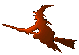Hex  1.0 Hydrogen-electron collision solver
Hex Documentation
Date
1. 2. 2014

# Hex-dwba

Hex-dwba is a high-energy computational module of the Hex package. It implements the distorted wave Born approximation of the first order and is expected to give trustworthy results for ehergies above 1 keV.

## Theory

The distorted wave Born approximation of the first order (DWBA-1) is similar to the well-known plane wave Born approximation. However, to speed up the convergence of the Born series (of which we are using just a single term in the first-order computation), part of the potential is excluded from the perurbative description and solved precisely. The spliting is the following

 $V = W + U \,$ (1)

where $$V$$ is the full potential felt by the projectile, $$U$$ is the so called "distorting potential" that will be solved precisely as mentioned above and explained below. This splitting has two consequences:

• First of all, the free states are no longer free, so we cannot use the plane waves. Instead, one receives a distorted waves as a solution of the modified equation for partial waves  $\left( -\frac{1}{2}\frac{\mathrm{d}^2}{\mathrm{d}r^2} +\frac{\ell(\ell+1)}{2r^2} +U(r) \right)\chi_{k\ell}(r) = E_{k}\chi_{k\ell}(r) \ .$ (2)
• Secondly, the T-matrix receives two contributions now – one from the scattering term and one as a correction due to the redefinition of the potentials. The result is the two-potential formula  $T = (N+1) \left<\chi_f^{(-)}\psi_f\right|V-U_f\left|\mathcal{A}\Psi_i^{(+)}\right> + \left<\chi_f^{(-)}\psi_f\right|U_f\left|\psi_i\beta_i\right> \ .$ (3)

## Implementation

The program iterates over partial waves of the asymptotic final state. For every iterations is will loop over all initial states that can contribute to the requested scattering process and still result in the current asymptotic final state.

The radial functions $$\chi_{k\ell}(r)$$ are computed using numerical solution of the equation (2) by the adaptive Cash-Karp Runge-Kutta method provided by GSL (see below).

## Requirements

You will need a modern C++ compiler to compile the program as for example

• GCC 4.8.1 or
• Intel C++ Composer XE 14.0 SP1 Update 1 (tested with GCC 4.8.1 headers)

DWBA uses some free external libraries

• GNU Scientific Library (1.16): for Wigner coupling coefficients, numerical integration and some other special functions.
• HDF5 (1.8.11): for standardized binary output of intermediate results (used to speed up further simillar calculations); optional.
• FFTW (3.3.3): for fast evaluation of Chebyshev expansions by Fast Fourier Transform.

The first is used for numerical integration and special and utitlity functions. The second is used for large-precision rational numbers that are necessary when working with analytic formulas. Both libraries are open-source and maintained. They can be downloaded free of charge and compiled on any Posix-compatible system. The compilation in Windows system can be done e.g. in the Code::Blocks IDE (that is not at all straightforward, though).